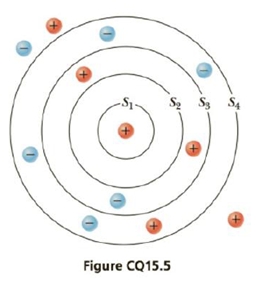Chapter 15, Problem 5CQ

Chapter
Section
Textbook Problem

Four concentric spheres S1, S2, S3, and S4 are arranged as in Figure CQ15.5 and each charge in the figure has the same magnitude. What is the ratio of the electric flux through spheres S2, S3, and S4 to the flux through sphere S1: (a) Φ2/Φ1 = _________ (b) Φ3/Φ1 = ___________ (c) Φ4/Φ1 = ________?(a)

To determine
The ratio of electric flux through spheres S2 and S1 .

Explanation

The electric flux is proportional to the charge enclosed ( qinside ) by the surface.

ϕqinside

Therefore,

ϕ2ϕ1=q2q1 (I)

• ϕ2 and ϕ1 are the electric flux of spheres S2 and S1 .
• q2 and q1 are the charges enclosed by spheres S2 and S1

(b)

To determine
The ratio of electric flux through spheres S3 and S1 .

(c)

To determine
The ratio of electric flux through spheres S4 and S1 .

Still sussing out bartleby?

Check out a sample textbook solution.

See a sample solution

The Solution to Your Study Problems

Bartleby provides explanations to thousands of textbook problems written by our experts, many with advanced degrees!

Get Started